# First week of activity at the Simons Institute

This first week at the Simons Institute was a lot of fun! I attended the first workshop in the Real Analysis program which was about Testing, Learning and Inapproximability. There was plenty of good talks and I learned a lot of new things.

– First I learned about parallel repetition. Letbe a finite alphabet. Consider a bipartite graphwith vertex sets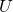andand a labeling of the edges with mappings fromto, that is edge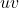is labelled with some mapping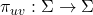. Now consider the following two players game (Alice and Bob are the players). First an edgeis drawn at random from some known probability distribution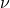over the set of edges in(the graphand the ‘labels’ are also known to the players). The vertex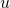is revealed to Alice and the vertex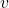is revealed to Bob. Without communication each one of them has to output an alphabet symbol, say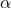for Alice and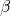for Bob, and they win if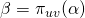. In other words they win if the pair of symbols is consistent with the labeling of the drawn edge. The value of the game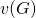is the probability that Alice and Bob wins, which can be rewritten as:Now we define the value of the repeated game as follows: the players simply play the above gametimes (with no communication at all except before the game starts when they agree on their strategy, and with the sequence of edges being i.i.d.) and the value of the repeated game, denoted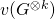is the probability that they win all thegames. This can be rewritten as:One is now interested in understanding the relation betweenand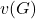.

At this point it’s probably a good idea to pause. If this is the first time that you read about parallel repetition you are probably like ‘wtf, it’s obvious that!!!’ (at least this was my initial reaction). It turns out that this is very far from being true. Clearly it’s true thatsince you can simply play thegames independently. But in some casesmight be much larger than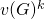, which is kind of amazing! Here is a beautiful example (which is due to Lance Fortnow I think) where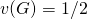and. The graphis the complete graph on the set of vertices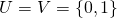and the distributionis uniform on the set of edges (in other words Alice receives a random bitand Bob receives a random bit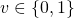). The alphabet is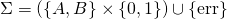where the symbolis introduced for sake of simplicity and we assume that the players cannot respond with. Now the mappingsare given by:In words the players must choose either Alice or Bob and then they both have to output the bit of the chosen player. For instance if they choose Alice then Bob has to guess the bit of Alice (and Alice can just report her bit). Clearly there is not much one can do for the one-shot game: one has. Now the situation becomes more interesting for the two rounds game: the players can agree that in the first round Bob will try to guess Alice’s first bit, and in the second round Alice will try to guess Bob’s second bit. The trick is that when Bob tries to guess Alice’s first bit he can use his second bit as a guess, and respectively Alice’s guess for Bob’s second bit can be her own first bit. That way they effectively reduced the two rounds game to a one-round game, since both predictions are correct if Bob’s second bit is equal to Alice’s first bit which happens with probability. In other words we proved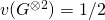while naively one would have guessed that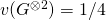.

Now that we have a better understanding of why the original question on the relation betweenandis a non-trivial one, it is clear that the interesting thing to study is to upper bound the valueas a function of(recall the obvious lower bound). In essence we are asking to show a limit to the power of tricks such as the one described above. Such a result was first proved by Ran Raz in 1995. He showed that, essentially, ifthen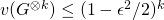(the original proof gaveinstead of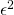). At the Simons workshop David Steurer talked about a new proof of this result based on viewing the value of the game as the norm of some matrix, see this paper. This new proof method also lead to new upper bounds, including(which matches some known lower bounds from Ran Raz). As far as I understand there are still plenty of open questions on this fundamental problem of parallel repetition.

– Joe Neeman talked about a new proof of Borell’s theorem which states that if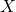andare two standard Gaussian vectors in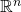with, then half-spaces are maximizers of the function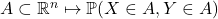. The proof is really slick, it’s basically one page of fairly simple calculations, see Section 2 hereElchanan Mossel then talked about the ‘natural’ extension of this result to the case of uniform variables on the hypercube, see this paper.

James Lee talked about a very interesting problem: we know how to prove lower bounds for the size of extended formulations (see this blog post for instance), but such bounds are interesting only for exact optimization. A natural question is whether we can prove lower bounds on the size of LPs even for approximate optimization. Apparently there is a rich literature on this for specific type of LP relaxations such the Sherali-Adams hierarchy. Now in their new paper (which is not yet online) James Lee and his co-authors are able to prove general bounds instead of the one that were previously proved specifically for the Sherali-Adams hierarchy. Of course LPs are nice but SDPs are even nicer (see here) and a natural question is how to generalize these results to SDPs instead of LPs. This was touched upon in Raghu Meka‘s talk where he discussed lower bounds for the number of rounds to solve the hidden clique problem with the Lasserre hierarchy. The paper, joint work with Avi Wigderson, is available here.

Nati Linial gave an absolutely wonderful talk, probably one of the best that I have seen in months. My advice is to look at the video as soon as it is released on the Simons website (this should be a link to it hopefully). Just as a teaser here is one object that Linial is looking at. First a definition: for a graphletbe the fraction of the set of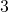vertices that contains exactly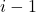edges. The vectoris called the-profile of. Now the object that we look at is the setof possible ‘asymptotic profile’, more precisely:There is not much that we know about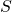. It is not convex: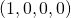andare clearly inbutis of course not in. We also know since the 60’s that for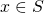one hasand Linial recently proved that. A better understanding of the properties ofcould lead to breakthroughs in the emerging theory of statistics for networks (see Nati’s talk for more details!).

Tomorrow the Big Data program will start with its Boot Camp. Apparently there has been a lot of hype around this boot camp and the seats have been ‘sold out’. If you can’t enter the auditorium, or if you are not in Berkeley, the event will be streamed live here.

This entry was posted in Theoretical Computer Science. Bookmark the permalink.

## 4 Responses to "First week of activity at the Simons Institute"

••••